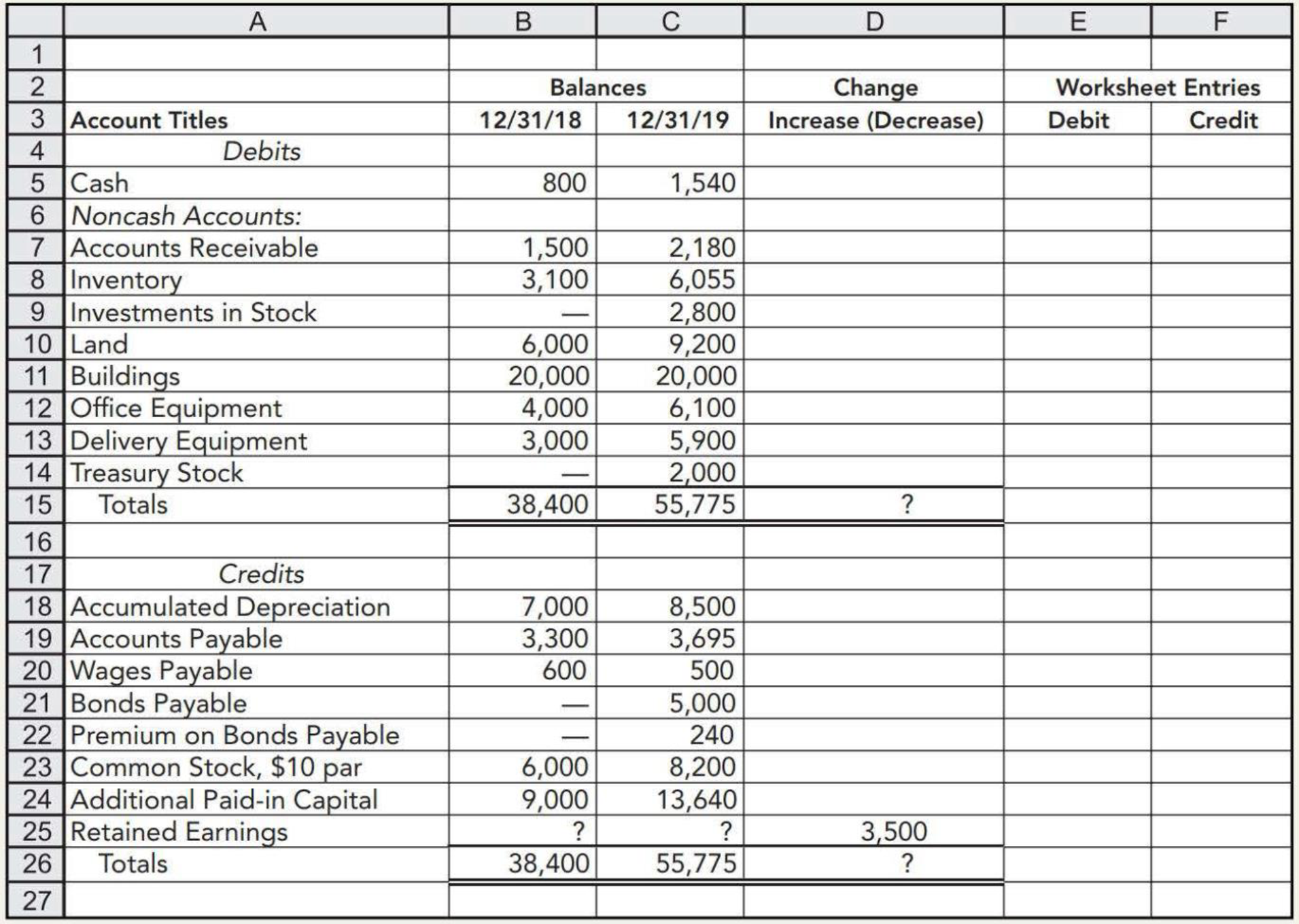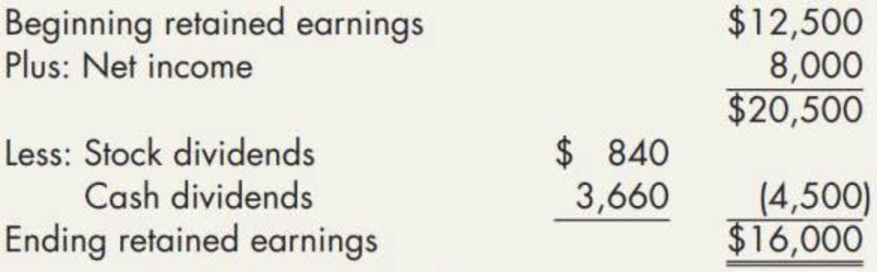Chapter 21, Problem 5P### Intermediate Accounting: Reporting...

3rd Edition
James M. Wahlen + 2 others
ISBN: 9781337788281

#### Solutions

Chapter
Section### Intermediate Accounting: Reporting...

3rd Edition
James M. Wahlen + 2 others
ISBN: 9781337788281
Textbook Problem
61 views

# Partially Completed Spreadsheet The following partially completed spreadsheet has been prepared for Perrin Company’s 2019 statement of cash flows:Additional relevant information:a. Accumulated depreciation is a contra account for all the depreciable assets. Depreciation on these assets totaled $2,200 for the year. b. On January 1, 2019, the company issued 10% bonds with a face value of$5,000 at 106. Interest was paid semiannually on June 30 and December 31. The bonds mature on January 1, 2024. Straight-line amortization is used for bond discount or premium. Bond interest expense was $440. c. Land was purchased for$3,200 during the year. d. Two hundred shares of common stock were issued for delivery equipment valued at $2,900 and office equipment valued at$3,100. e. Twenty shares of stock were issued as a stock dividend. The market price per share was $42. f. Office equipment with a cost of$1,000 and a book value of $300 was sold for$50. g. Fifty shares of its own common stock were reacquired by the company as treasury stock. The company purchased the shares for $40 per share. h. One hundred shares of Doe Company stock were purchased for$28 per share at year-end. Required:Complete the spreadsheet.

To determine

Prepare the completed spreadsheet of P Company for the year 2019.

Explanation

Worksheet: A worksheet is a spreadsheet used while preparing a financial statement. It is a type of form having multiple columns and it is used in the adjustment process. The use of a worksheet is optional for any organization. A worksheet can neither be considered as a journal nor a part of the general ledger.

Table (1)

Table (2)

Working notes:

a) Net income for the year 2019 is $8,000. b) Depreciation on the assets for the year 2019 is$2,200.

c) Calculate the increase in accounts receivable.

Increase in accounts receivable =(Closing accounts receivale Opening accounts receivable)=$2,180$1,500=$680 d) Calculate an increase in inventory. Increase in inventory = (Closing inventoryOpening inventory)=$6,055$3,100=$2,955

e) Calculate the increase in accounts payable.

Increase in accounts payable=(Closing balance of accounts payableOpening balance of accounts payable)=$3,695$3,300=$395 f) Calculate the decrease in wages payable. Decrease in wages payable = (Opening balance of wages payable Closing balance of wages payable)=$600$500=$100

g) Calculate proceeds from issuance of bond

### Still sussing out bartleby?

Check out a sample textbook solution.

See a sample solution

#### The Solution to Your Study Problems

Bartleby provides explanations to thousands of textbook problems written by our experts, many with advanced degrees!

Get Started